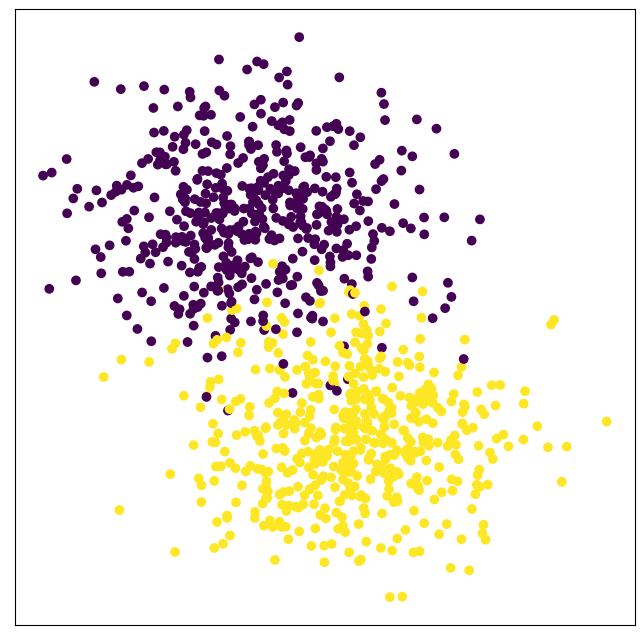# Outlier Detection

The outlier detection function of CLASSIX is fully dominated by the parameter of `minPts`. We have mentioned this parameter in the previous sections. However, if you would like to employ the outlier detection, we should set `post_alloc=False`, then the outliers will be marked as -1 instead of being allocated to other clusters. The example is as below:

Note

The minPts criterion is applied on the cluster level in CLASSIX, while in DBSCAN it is used to distinguish between noise, core, and boundary points.

```from sklearn import datasets
from classix import CLASSIX
import matplotlib.pyplot as plt
X, y = datasets.make_blobs(n_samples=1000, centers=2, n_features=2, random_state=0)
clx = CLASSIX(sorting='pca', radius=0.15, group_merging='density', verbose=1, minPts=13, post_alloc=False)
clx.fit(X)

plt.figure(figsize=(8,8))
plt.rcParams['axes.facecolor'] = 'white'
plt.scatter(X[:,0], X[:,1], c=clx.labels_)
plt.xticks([])
plt.yticks([])
plt.show()
```

The groud truth label is:The clustering result is:All outliers output by CLASSIX are marked as black. As shown in the figure, the outliers classified by CLASSIX are far away from the cluster center points.